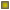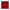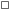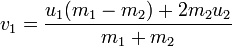# Elastic Collisions Inspiration...

date: 11 Apr, 2010, actionscript: 3 FP10, complexity:, feedback (votes):### Music - last records

Hi there,

Last weekend I had some spare time for writing an article I got in mind from long time ago, this is about Elastic Collisions, pretty much simple concept but apparently there are some people implementing it in the wrong way (I heard about some crazy exceptions, many bugs and problems people have) and in most of these cases it is because of they are detecting collisions in a wrong workflow. When we speak about elastic collisions we are speaking about the response of it after the collision happens and not when it actually happens (its detection), you must be careful in the way how you detect when the collisions occur, one think is detecting when the collision happens and other completely different is what happen after it.

First time I worked with elastic collisions was using actionscript 2, after many tests I did the performance of all these formulas were quite good due to its simplicity of how it works. The main concept is quite simple; an elastic collision is a collision between two objects (bodies which have mass) where the total momentum of the objects colliding are preserved. In other words, none of the energy involved in the collision is lost; it is distributed between the action objects depending on their mass and speed they got when the collision happened.

If you google about "elastic collisions" you may find thousands of articles and information around but unfortunately not all of this is correct, there are many people out there "as usual" explaining things in the most complicated way. I didn’t find any simple website where we can find out and understand about it easily or maybe I wasn’t lucky enough. I recommend to you take a look on the content published in Wikipedia if you want to know a bit more about it, it is really simple and well explained over there, they tell you how it works and also covers some aspects of 2 and 3 dimension collisions, the link is Elastic collision, note that the elastic collision formulas always deal with the response once the collision happens, you have to detect when the collision happen by yourself which is not hard but can be tricky to sort out when you’re facing many objects colliding at the same time.

Anyway the formula applied in one dimension (linear) is pretty much this:andWait!! Don’t run away! It is so simple to interpret it, basically what it says is; if we have two objects A and B and the object A has a mass of 3 Kilograms and it is moving with a speed of 1 meter/sec and the Object B which has a mass of 1 Kilogram is moving at 2 meters/sec, What will happen when these two objects collide? Which are the new forces (speed) for each? easy...

mass1 = 3 //mass of object A
vel1 = -1 //speed of object A (negative because of collision, opposite direction against object B)
mass2 = 1 //mass of object B
vel2 = 2 //speed of object B
bothmass = mass1 + mass2
newvel1 = (vel1 * (mass1 - mass2) + (2 * mass2 * vel2)) / bothmass
newvel2 = (vel2 * (mass2 - mass1) + (2 * mass1 * vel1)) / bothmass
//result...
//newvel1 = 0.5
//newvel2 = 2.5

That’s all you need to know about it. Departing from this point I have made the following example using Flash Develop and the Open Source Flex SDK, the source of it is here download sourcecode. This example shows how the elastic collision can be implemented in flash in a very, very simple way; the whole program doesn’t have more than 30 instructions, I think that it really worth to have a go with it.

And then here is something interesting I found by chance at the science museum in London one day I was walking nearby and I decided to take a quick look inside. I met a machine that made me come back later with my camera and make a short video of it. This is a short video (no longer than 2 minutes) but I think enough for explaining what it is about and its connection with elastic collisions. After you watch it, you will be able to understand what the next experiment is about:

Well, when I saw that crazy machine (Energy ring by soda.co.uk) the first thing that came to my mind was if it was actually using elastic collision or not, and I was wondering if it is possible to simulate its behaviour in flash using simply elastic collisions. My approach on this experiment is totally oriented to the movement algorithm and the collision between the blocks you can see on the video, so things like the small particles flying around and the text on the display panel are not begin treated here, this is 100% oriented to the collision behaviour applying some gravity on the objects inside a circular path, I hope you see my point. Here is the sourcecode if you want to take a look on it. I think this source code is pretty much the same of the previous example apart of moving objects in a circle rather than on the x axis and colliding between these two limits (the walls), here they just collide between them and also I’m applying gravity taking advantage of the sine curve. Does it look similar to the video? I think that it is quite much what we can achieve using elastic collisions.

## What about 2D elastic collisions?

Another approach of this elastic collision concept could be the collisions between balls, think about a billar pool game, the collision between the balls of a pool game is pretty much an authentic elastic collision with some extra factors that makes everything harder to achieve when coding a game such as; the friction of balls with air and on the table surface plus the spinning effect that they could have when moving that alters completely the collision response. I've been working long time ago on a game that unfortunately I never got the chance of finish. It is about an "8 ball" pool game using 15 balls and handling elastic collisions between them (without ball effects). So, here is what I have done so far, in this unfinished version I’m not making any validations about the game, it is just a free table all the time, hit what ever you want and then if put the white ball in a pocket I just put it back to the table behind the line. Unfortunately the source code is quite old, rusty and messy and is not available for downloading but I promise it will be as soon as I have a chance to tidy it up a bit. Take a look on it.

When we are speaking about physics, may be the most important thing about it, is to know when things exactly happen. It is quite important detect collisions in the right way, fast (in terms of performance) and highly responsible in terms of accuracy. The way how I'm detecting the balls collisions in the previous experiment is using the following function that by the way It doesn't use Math.sin and Math.cos functions at all which makes everything runs much faster.

static public function findTimeUntilCollide(b1:Ball, b2:Ball):Number {
//note that Ball properties vx and vy are the speed in x and y axis.
var collision_time:Number = -1;
// Compute parts of quadratic formula
// a = (v2x - v1x) ^ 2 + (v2y - v1y) ^ 2
var a:Number = Math.pow(b2.vx - b1.vx, 2) + Math.pow(b2.vy - b1.vy, 2);
// b = 2 * ((x20 - x10) * (v2x - v1x) + (y20 - y10) * (v2y - v1y))
var b:Number = 2. * ((b2.x - b1.x) * (b2.vx - b1.vx) + (b2.y - b1.y) * (b2.vy - b1.vy));
// c = (x20 - x10) ^ 2 + (y20 - y10) ^ 2 - (r1 + r2) ^ 2
var c:Number = Math.pow(b2.x - b1.x, 2) + Math.pow(b2.y - b1.y, 2) - Math.pow(b1.r + b2.r, 2);
// Determinant = b^2 - 4ac
var det:Number = Math.pow(b,2) - (4 * a * c);
if (a != 0.) { // If a == 0 then v2x==v1x and v2y==v1y and there will be no collision
var t:Number = (-b - Math.sqrt(det)) / (2. * a); // Quadratic formula. t = time to collision
if (t >= 0.) { // If collision occurs...
collision_time = t;
}
}
return collision_time; //if it is less or equal 1 it means that will be in contact before next frame
}

Here is this function in practice... drag and drop components to find out how does it work.

And then if you’re wondering what the response of the collision using elastic collisions is, here is the function I used for it and also for predicting the white ball path before you hit it:

static public function doElasticCollision(b1:Ball,b2:Ball){
// Compute unit normal and unit tangent vectors
// v_n = normal vec. - a vector normal to the collision surface
var v_n:Point = new Point(b2.x-b1.x, b2.y-b1.y);
var hyp:Number = getHypotenuse(v_n.x,v_n.y); //distance between dots
var v_un:Point = new Point(v_n.x/hyp, v_n.y/hyp); // unit normal vector
var v_ut:Point = new Point(-v_un.y, v_un.x); // unit tangent vector
// Compute scalar projections of velocities onto v_un and v_ut
var v1n:Number = v_un.x * b1.vx + v_un.y * b1.vy;
var v1tPrime:Number = v_ut.x * b1.vx + v_ut.y * b1.vy;
var v2n:Number = v_un.x * b2.vx + v_un.y * b2.vy;
var v2tPrime:Number = v_ut.x * b2.vx + v_ut.y * b2.vy;
// Compute new velocities using one-dimensional elastic collision equations in the normal direction
// Division by zero avoided. See early return above.
var v1nPrime:Number = (v1n * (b1.m - b2.m) + 2. * b2.m * v2n) / (b1.m + b2.m);
var v2nPrime:Number = (v2n * (b2.m - b1.m) + 2. * b1.m * v1n) / (b1.m + b2.m);
// Compute new normal and tangential velocity vectors
var v_v1nPrime:Point = new Point(v_un.x*v1nPrime, v_un.y*v1nPrime);
var v_v1tPrime:Point = new Point(v_ut.x*v1tPrime, v_ut.y*v1tPrime);
var v_v2nPrime:Point = new Point(v_un.x*v2nPrime, v_un.y*v2nPrime);
var v_v2tPrime:Point = new Point(v_ut.x*v2tPrime, v_ut.y*v2tPrime);
//update velocities.
b1.vx = v_v1nPrime.x + v_v1tPrime.x;
b1.vy = v_v1nPrime.y + v_v1tPrime.y;
b2.vx = v_v2nPrime.x + v_v2tPrime.x;
b2.vy = v_v2nPrime.y + v_v2tPrime.y;
}

These functions are quite fast in term of performance, they don’t use Math.sin and Math.cos at all, I hope this article is useful to someone, it took me ages of research to find out all this information you can find here, if you use some of this code don't forget to make a donation (a beer), cheers!

Totally Awesome! I have been playing for hours. You create amazing pieces of "art"
newvel2 = (vel2 * (mass2 - mass1) + (2 * mass1 * vel1)) / bothmass result newvel2 = (it is -2.5)
Yes, It is in my todo list, I will publish it as soon as I have a chance, I need to finish that game and then tidy up that code a bit, put some comments on it about the critical areas, I think the most important parts of it are already metionated in this article, the algorithms. Anyway, I will... cheers!
Could you please post the source files for the billiards game?
yes it is a problem of my algorithm, and it is the reason why i have my balls very very big on stage :D everyone looks suprised by the size of them. but i get the point, if i write another elastic colision function, it will definitely be different :) thanx bro!
Hi Tarik Kaya. Well, I will answer your question with another question, in your system, What does happen if two balls are far separated and they are moving quite fast against each other? So from frame one to two the position of the ball A will be the position of B and viceversa. Interception never happens? So let me guess, the collision never occurs? When dealing with physics the most important thing is to know when things happen and things like when them overlaps is too late.
nice work! i appreciate it. I have a question for you, i started to write a billard game myself and it is almost finished.
but the physics i wrote works not like yours but this way(which seemed much easier at the start, but then i understood that i screwed up :D ):
nice work! i appreciate it. I have a question for you, i started to write a billard game myself and it is almost finished.
i am not predicting when the balls will collide, but instead in each frame, checking if any balls are inside each other.
if some two balls are inside each other i take them out of each other, by taking them back according to their earlier speeds. after that i have it all handled. I mean my elastic collision works, and it might be better than yours, it seemed to me. (i can share if you would like). but my problem is at the collision prediction...